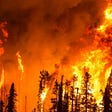# Building Deep Autoencoders with Keras and TensorFlow

In this tutorial, we will explore how to build and train deep autoencoders using Keras and Tensorflow.

The primary reason I decided to write this tutorial is that most of the tutorials out there, including the official Keras and TensorFlow ones, use the MNIST data for the training. I have been asked numerous times to show how to train autoencoders using our own images that may be large in number.

I will try to keep this tutorial brief and will not get into the details of how autoencoder works. Therefore, having a basic knowledge of autoencoders is the prerequisite to understand the code presented in this tutorial (needless to say that you must know how to program in Python, Keras and TensorFlow).

# Autoencoders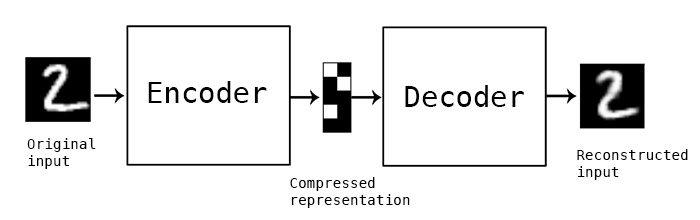Figure 1: A simplified visualization of autoencoders (image courtesy: Francois Chollet, https://blog.keras.io/building-autoencoders-in-keras.html)

Autoencoders are unsupervised neural networks that learn to reconstruct its input. Denoising an image is one of the uses of autoencoders. Denoising is very useful for OCR. Autoencoders are also also used for image compression.

As shown in Figure 1, an autoencoder consists of:

1. Encoder: The encoder takes an image as input and generates an output which is much smaller dimension compared to the original image. The output from the encoders is also called as the latent representation of the input image.
2. Decoder: The decoder takes the output from the encoder (aka the latent representation of the input image) and reconstructs the input image.

Both encoders and decoders are convolutional neural networks with the difference that the encoders dimensions reduce with each layer and the decoders dimensions increase with each layer until the output layer where the dimensions match with the original image.

# Training Autoencoders

We will use our own images for training and testing the autoencoders. For the purpose of this tutorial, we will use a dataset that contains scanned images of restaurant receipts. The dataset is freely available from the link https://expressexpense.com/large-receipt-image-dataset-SRD.zip uner MIT License.

Although this dataset does not have a large number of images, we will write code that will work for both small and large datasets.

The code below is divided into 4 parts.

1. Data preparation: Images will be read from a directory and fed as inputs to the encoder block.
2. Neural network configuration: We will write a function that takes certain parameters and return the encoder, decoder and autoencoder convolutional neural networks
3. Training the neural networks: The code that triggers the training, monitors the progress and saves the trained models.
4. Prediction: The code block that uses the trained models and predicts the output.

I will use Google Colaboratory (https://colab.research.google.com/) to execute the code. You can use your favorite IDE to write and run the code. The code below works both for CPUs and GPUs, I will use the GPU based machine to speed up the training. Google Colab offers a free GPU based virtual machine for education and learning.

If you use a Jupyter notebook, the steps below will look very similar.

First we create a notebook project, AE Demo for example.

Before we start the actual code, let’s import all dependencies that we need for our project. Here is a list of imports that we will need.

# Import the necessary packages

import tensorflow as tf

from tensorflow.keras.layers import BatchNormalization

from tensorflow.keras.layers import Conv2D

from tensorflow.keras.layers import Conv2DTranspose

from tensorflow.keras.layers import LeakyReLU

from tensorflow.keras.layers import Activation

from tensorflow.keras.layers import Flatten

from tensorflow.keras.layers import Dense

from tensorflow.keras.layers import Reshape

from tensorflow.keras.layers import Input

from tensorflow.keras.models import Model

from tensorflow.keras import backend as K

import numpy as np

Listing 1.1: Import the necessary packages.

# Data Preparation:

Our receipt images are in a directory. We will use ImageDataGenerator class, provided by Keras API, and create training and test iterators as shown in the listing 1.2 below.

trainig_img_dir = “inputs”

height = 1000

width = 500

channel = 1

batch_size = 8

datagen = tf.keras.preprocessing.image.ImageDataGenerator(validation_split=0.2, rescale=1. / 255.)

train_it = datagen.flow_from_directory(

trainig_img_dir,

target_size=(height, width),

color_mode=’grayscale’,

class_mode=’input’,

batch_size=batch_size,

subset=’training’) # set as training data

val_it = datagen.flow_from_directory(

trainig_img_dir,

target_size=(height, width),

color_mode=’grayscale’,

class_mode=’input’,

batch_size=batch_size,

subset=’validation’) # set as validation data

Listing 1.2: Image input preparation. Load images in batches from a directory.

1. training_img_dir = “inputs” is the parent directory that contains the receipt images. In other words, receipts are in a subdirectory under the “inputs” directory.
2. color_mode=’grayscale’ is important if you want to convert your input images into grayscale.

All other parameters are self explanatory.

# Configure Autoencoder Neural Networks

As shown in Listing 1.3 below, we have created an AutoencoderBuilder class that provides a function build_ae(). This function takes the following arguments:

• height of the input images,
• width of the input images,
• depth (or the number of channels) of the input images.
• filters as a tuple with the default as (32,64)
• latentDim which represents the dimension of the latent vector

class AutoencoderBuilder:

@staticmethod

def build_ae(height, width, depth, filters=(32, 64), latentDim=16):

#Initialize the input shape.

inputShape = (height, width, depth)

chanDim = -1

# define the input to the encoder

inputs = Input(shape=inputShape)

x = inputs

# loop over the filters

for filter in filters:

# Build network with Convolutional with RELU and BatchNormalization

x = Conv2D(filter, (3, 3), strides=2, padding=”same”)(x)

x = LeakyReLU(alpha=0.2)(x)

x = BatchNormalization(axis=chanDim)(x)

# flatten the network and then construct the latent vector

volumeSize = K.int_shape(x)

x = Flatten()(x)

latent = Dense(latentDim)(x)

# build the encoder model

encoder = Model(inputs, latent, name=”encoder”)

# We will now build the the decoder model which takes the output from the encoder as its inputs

latentInputs = Input(shape=(latentDim,))

x = Dense(np.prod(volumeSize[1:]))(latentInputs)

x = Reshape((volumeSize, volumeSize, volumeSize))(x)

# We will loop over the filters again but in the reverse order

for filter in filters[::-1]:

# In the decoder, we will apply a CONV_TRANSPOSE with RELU and BatchNormalization operation

x = Conv2DTranspose(filter, (3, 3), strides=2,

x = LeakyReLU(alpha=0.2)(x)

x = BatchNormalization(axis=chanDim)(x)

# Now, we want to recover the original depth of the image. For this, we apply a single CONV_TRANSPOSE layer

x = Conv2DTranspose(depth, (3, 3), padding=”same”)(x)

outputs = Activation(“sigmoid”)(x)

# Now build the decoder model

decoder = Model(latentInputs, outputs, name=”decoder”)

# Finally, the autoencoder is the encoder + decoder

autoencoder = Model(inputs, decoder(encoder(inputs)),

name=”autoencoder”)

# return a tuple of the encoder, decoder, and autoencoder models

return (encoder, decoder, autoencoder)

Listing 1.3: Builder class to create autoencoder networks.

# Training Autoencoders

The following code Listing 1.4 starts the autoencoder training.

# initialize the number of epochs to train for and batch size

EPOCHS = 300

BATCHES = 8

MODEL_OUT_DIR = “ae_model_dir”

# construct our convolutional autoencoder

print(“[INFO] building autoencoder…”)

(encoder, decoder, autoencoder) = AutoencoderBuilder().build_ae(height,width,channel)

autoencoder.compile(loss=”mse”, optimizer=opt)

# train the convolutional autoencoder

history = autoencoder.fit(

train_it,

validation_data=val_it,

epochs=EPOCHS,

batch_size=BATCHES)

autoencoder.save(MODEL_OUT_DIR+”/ae_model.h5”)

Listing 1.4: Training autoencoder model.

# Visualizing the Training Metrics

The code listing 1.5 shows how to display a graph of loss/accuracy per epoch of both training and validation. Figure 2 shows a sample output of the code Listing 1.5

# set the matplotlib backend so figures can be saved in the background

import matplotlib

import matplotlib.pyplot as plt

%matplotlib inline

# construct a plot that plots and displays the training history

N = np.arange(0, EPOCHS)

plt.style.use(“ggplot”)

plt.figure()

plt.plot(N, history.history[“loss”], label=”train_loss”)

plt.plot(N, history.history[“val_loss”], label=”val_loss”)

plt.title(“Training Loss and Accuracy”)

plt.xlabel(“Epoch #”)

plt.ylabel(“Loss/Accuracy”)

plt.legend(loc=”lower left”)

# plt.savefig(plot)

plt.show(block=True)

Listing 1.5: Display a plot of training loss and accuracy vs epochs

Figure 1.2: Plot of loss/accuracy vs epoch

# Make Predictions

Now that we have a trained autoencoder model, we will use it to make predictions. The code listing 1.6 shows how to load the model from the directory location where it was saved. We use predict() function and pass the validation image iterator that we created before. Ideally we should have a different image set for prediction and testing.

Here is the code to do the prediction and display.

# use the convolutional autoencoder to make predictions on the

# validation images, then display those predicted image.

print(“[INFO] making predictions…”)

decoded = autoencoder_model.predict(train_it)

decoded = autoencoder.predict(val_it)

examples = 10

# loop over a few samples to display the predicted images

for i in range(0, examples):

predicted = (decoded[i] * 255).astype(“uint8”)

cv2_imshow(predicted)

Listing 1.6: Code to predict and display the images

In the above code listing, I have used the cv2_imshow package which is very specific to Google Colab. If you are Jupyter or any other IDE, you may have to simply import the cv2 package. To display the image, use cv2.imshow() function.

# Conclusion

In this tutorial, we built autoencoder models using our own images. We also explored how to save the model. We loaded the saved model and made the predictions. We finally displayed the predicted images.

--

--

--

## More from Building Deep Autoencoder with Keras and TensorFlow

This hands-on tutorial shows with code examples of how to train autoencoders using your own images.

## Glow: Graph Lowering Compiler Techniques for Neural Network## Decision Trees## NLP with graphs on WeDeploy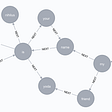## How to ___ Variational AutoEncoder ?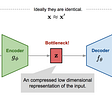## Super Musician: Music Style Transfer Based on AutoEncoder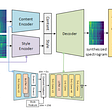## Machine Learning integration with DevOps...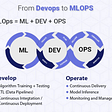## Sam Ansari

Author, inventor and thought leader in computer vision, machine learning, and AI. 4 US Patents.

## Preventing robberies in urban environments using deep learning and OpenCV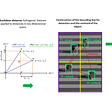## The Experiment of Forest Fires Prediction using Deep Learning